3SL Reference

Welcome to the 3SL Reference Section

This section of the website explains what systems engineering is, how various different methodologies work, where requirements management, model based systems engineering, traceability, assessing risks, testing and lots of other elements come together to ensure projects stay manageable, costly, safe and deliverable.

This section will also show how various modelling systems work in Cradle and demonstrate how systems engineering projects can be managed, from concept to creation, with this single end-to-end tool.

This section will be developed on an on-going basis.

Needs in Systems Engineering

Needs in Systems Engineering discusses the role and representation of different types of systems engineering information in any agile or phase-based process in organisations that produce products.

User Requirements in Systems Engineering

User Requirements in Systems Engineering describes how user requirements should be derived from stakeholders’ statements, structured in a Cradle database, and used to engineer products

Model-Based Systems Engineering (MBSE)

Model-Based Systems Engineering (MBSE) is a Systems Engineering foundation which highlights the application of visual modelling principles and best practice to Systems Engineering activities throughout the development lifecycle. Such activities include requirements management, requirements analysis, validation & verification, functional analysis, performance analysis and system architecture definition and specification.

Below are examples of how Cradle utilises models for many different kinds of Systems Engineering applications.

This MBSE section is split into three sections to demonstrate the three licensing types of Systems Modelling Cradle can perform. SysML, UML and Functional Architecture Data Modelling (Classic Modelling).

SysML Modelling: Activity Diagram (act)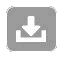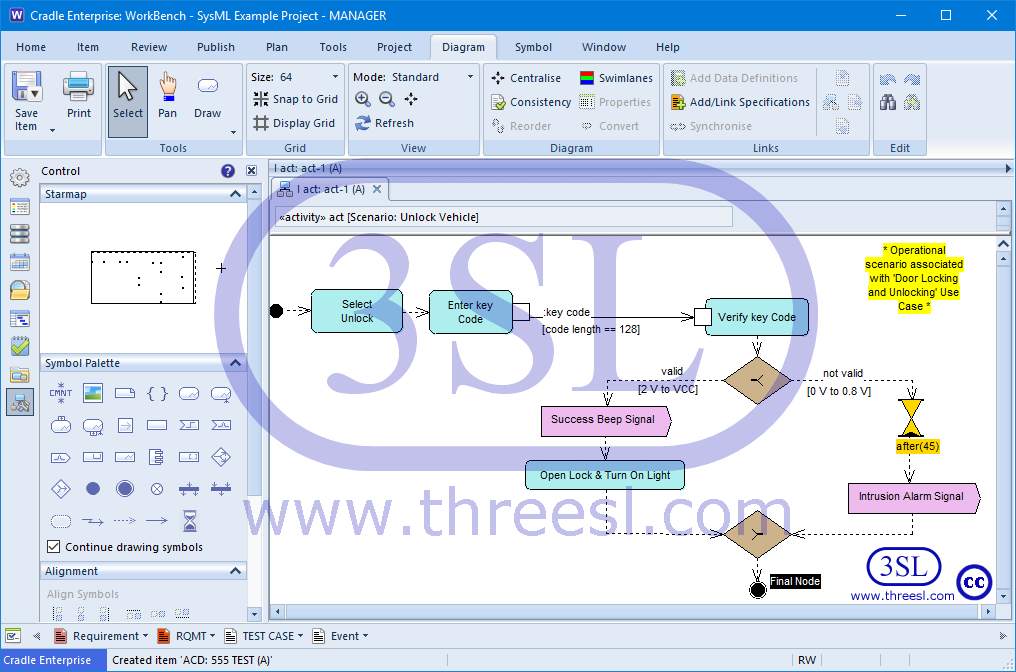Activity Diagram (act)

SysML Modelling: Block Definition Diagram (bdd)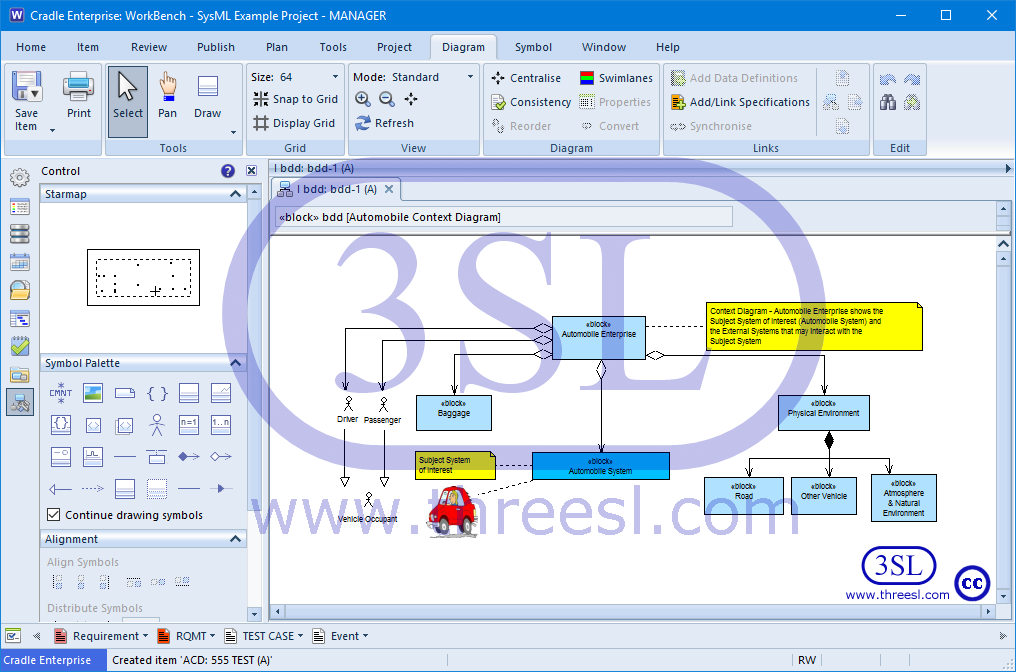Block Definition Diagram (bdd)

SysML Modelling: Internal Block Diagram (ibd)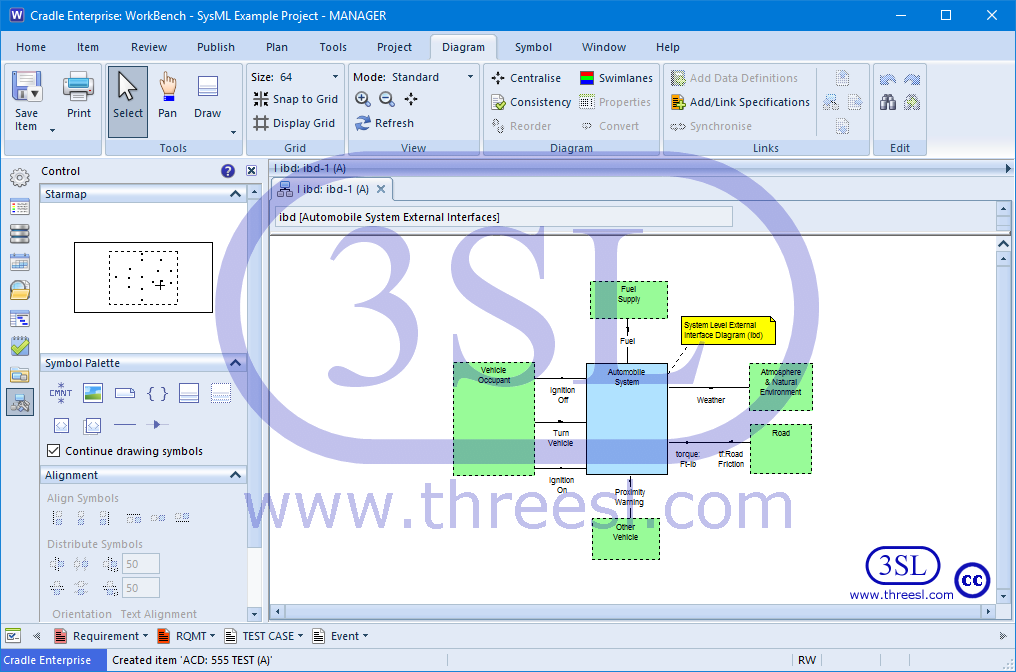Internal Block Diagram (ibd)

SysML Modelling: Package Diagram (pkg)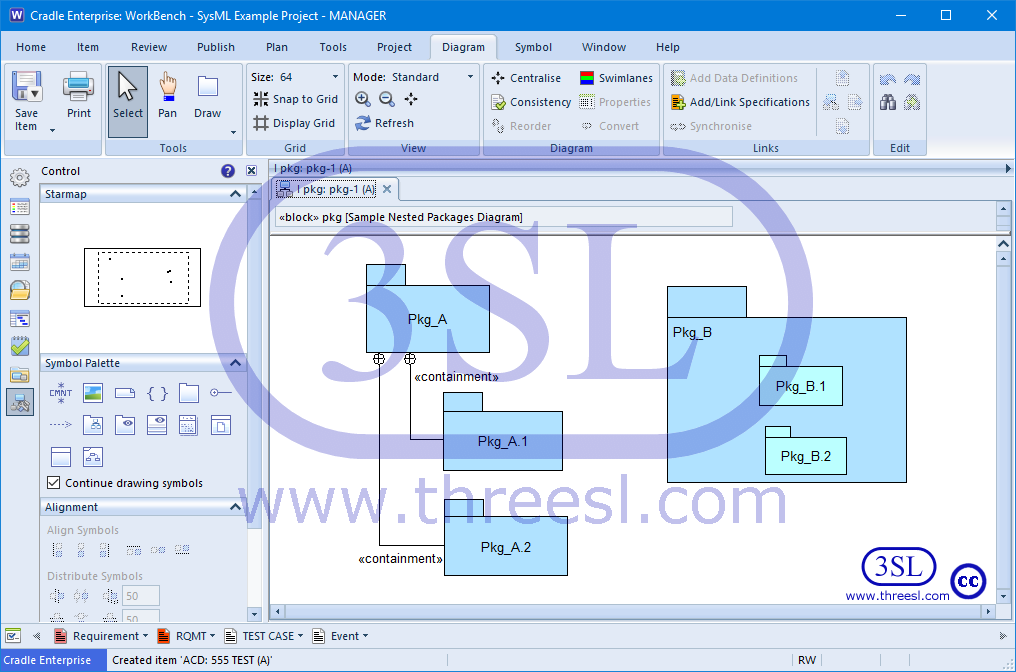Package Diagram (pkg)

SysML Modelling: Parametric Diagram (par)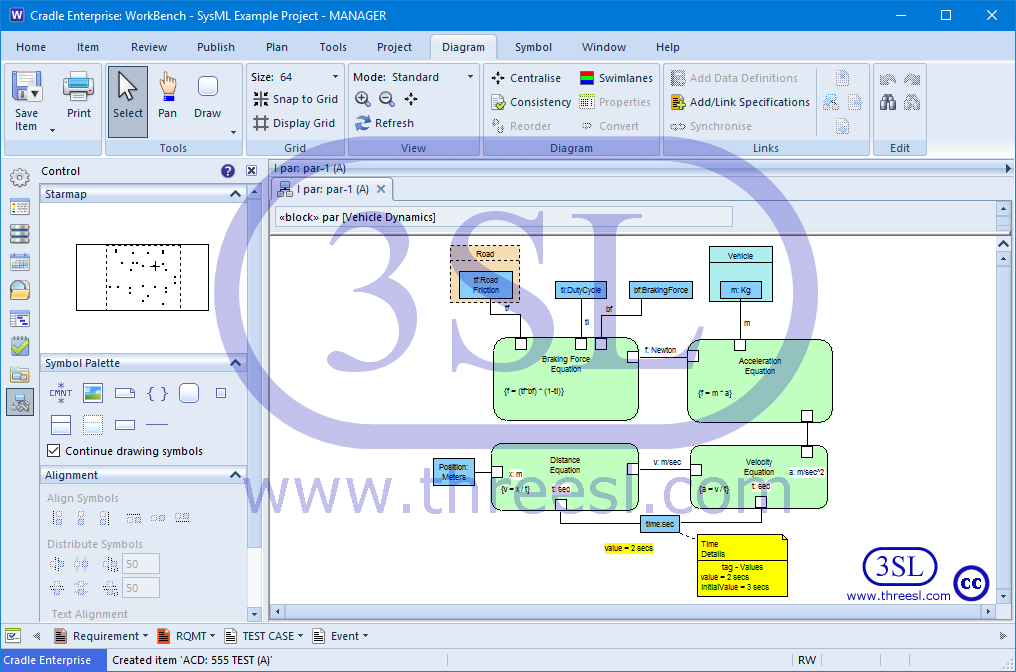Parametric Diagram (par)

SysML Modelling: Sequence Diagram (sd)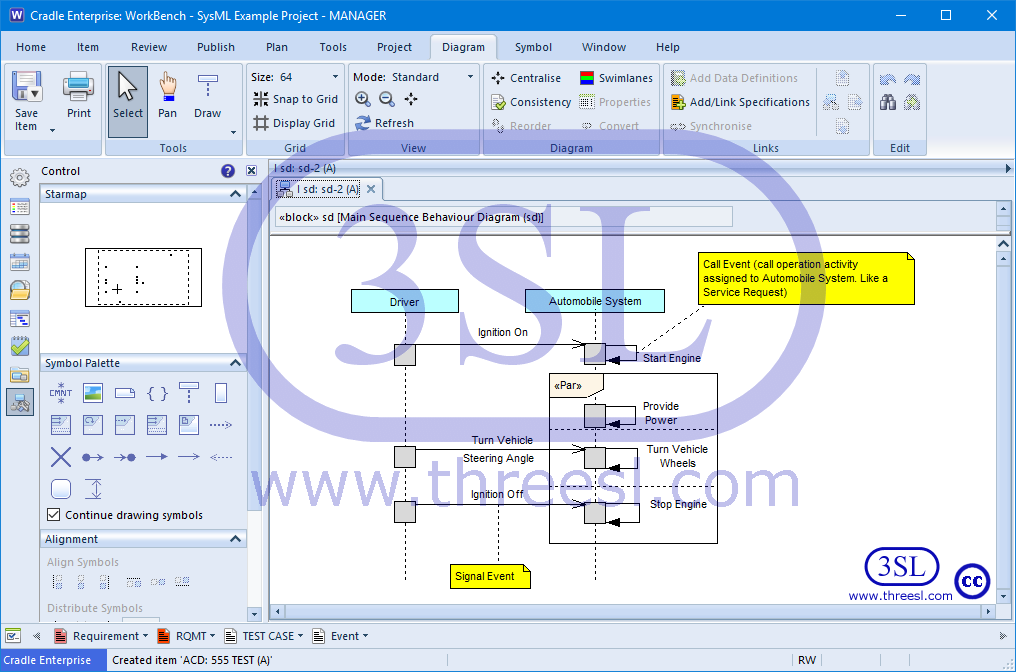Sequence Diagram (sd)

SysML Modelling: Requirement Diagram (req)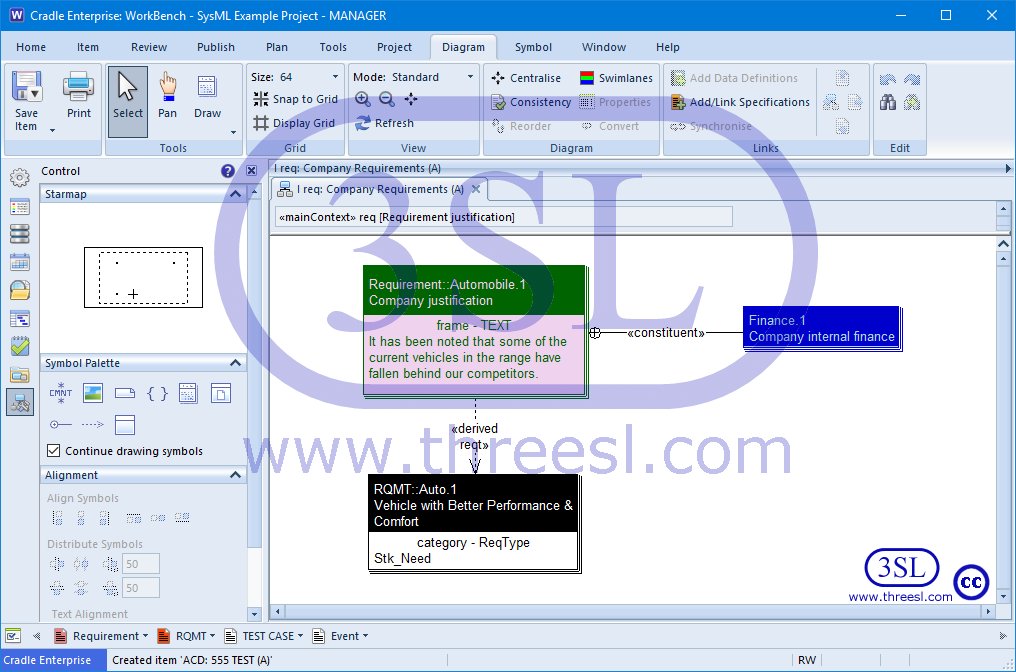Requirement Diagram (req)

SysML Modelling: State Machine Diagram (stm)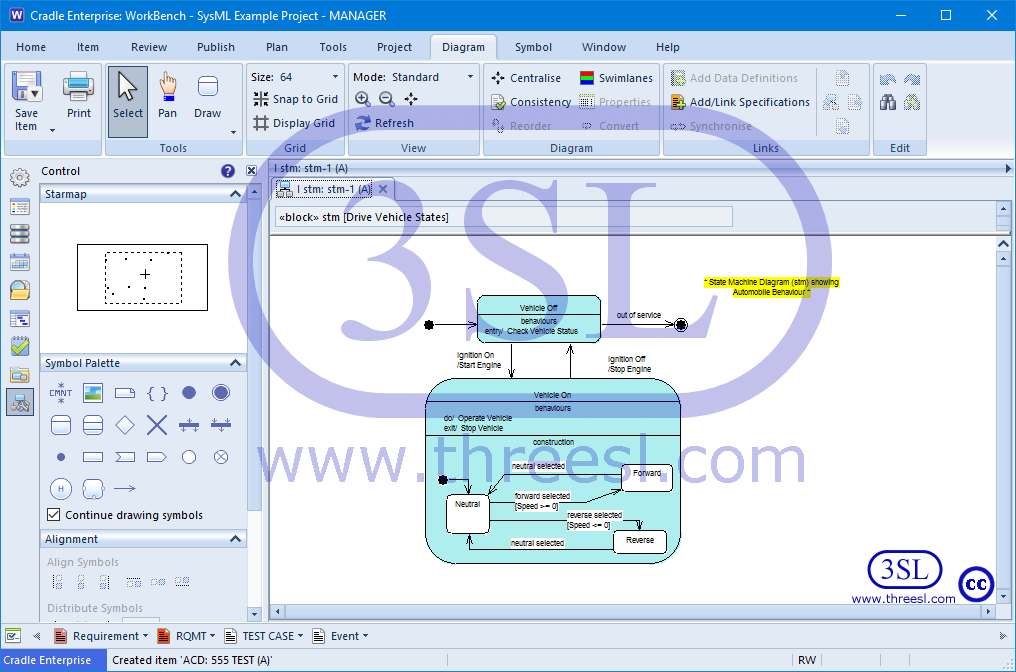State Machine Diagram (stm)

SysML Modelling: Use Case Diagram (uc)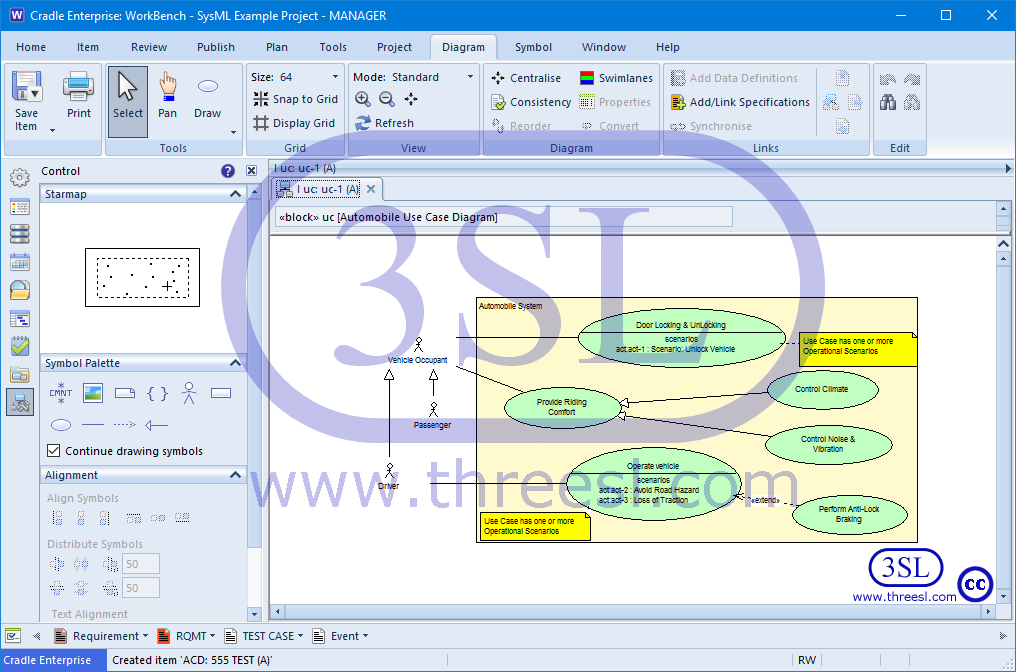Use Case Diagram (uc)

Functional Modelling: Data Flow Diagrams (DFD)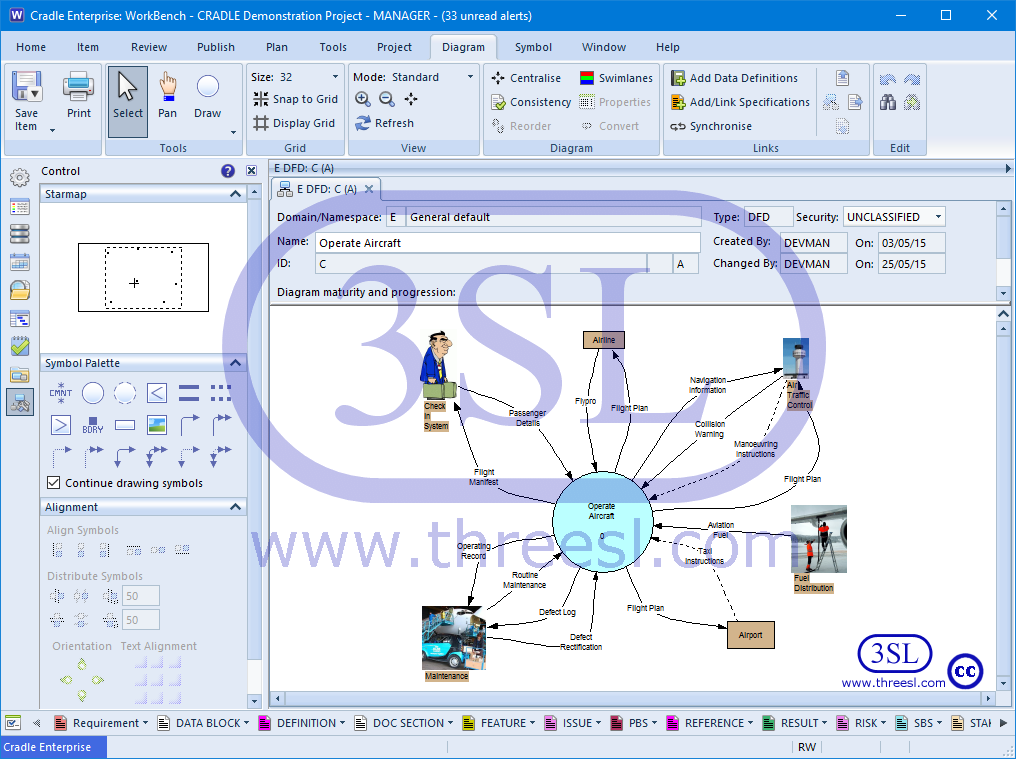Data Flow Diagrams (DFD)

Functional Modelling: State Transition Diagram (STD)State Transition Diagram (STD)

Functional Modelling: Structure Chart (STC)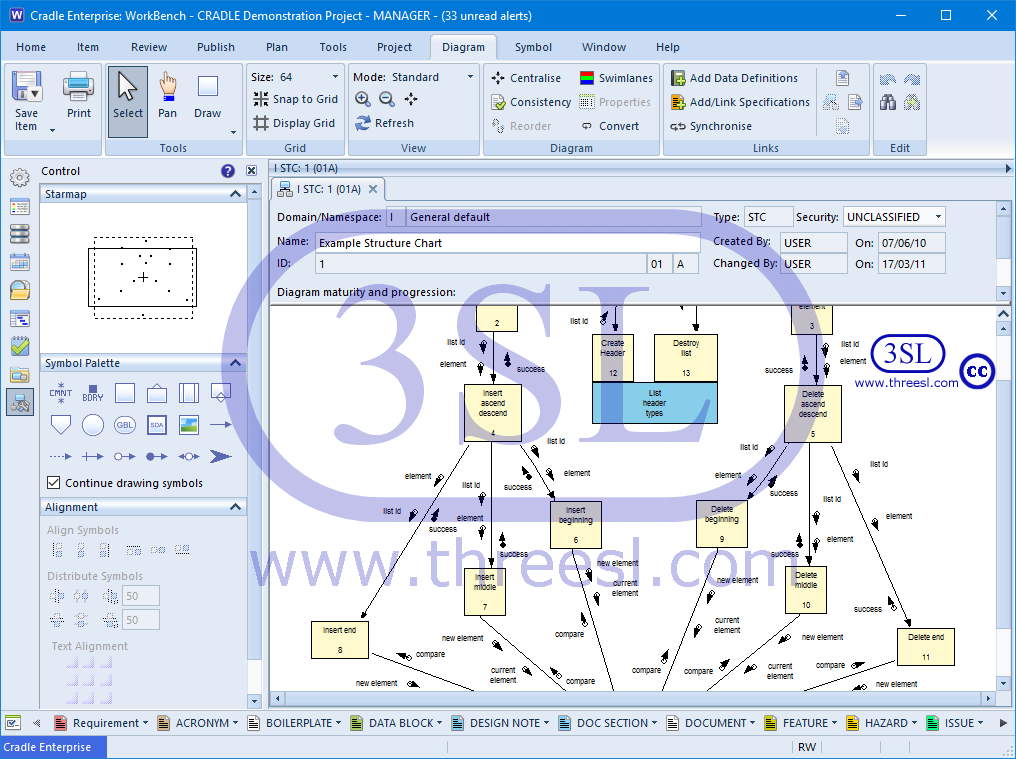Structure Chart (STC)

Functional Modelling: IDEF0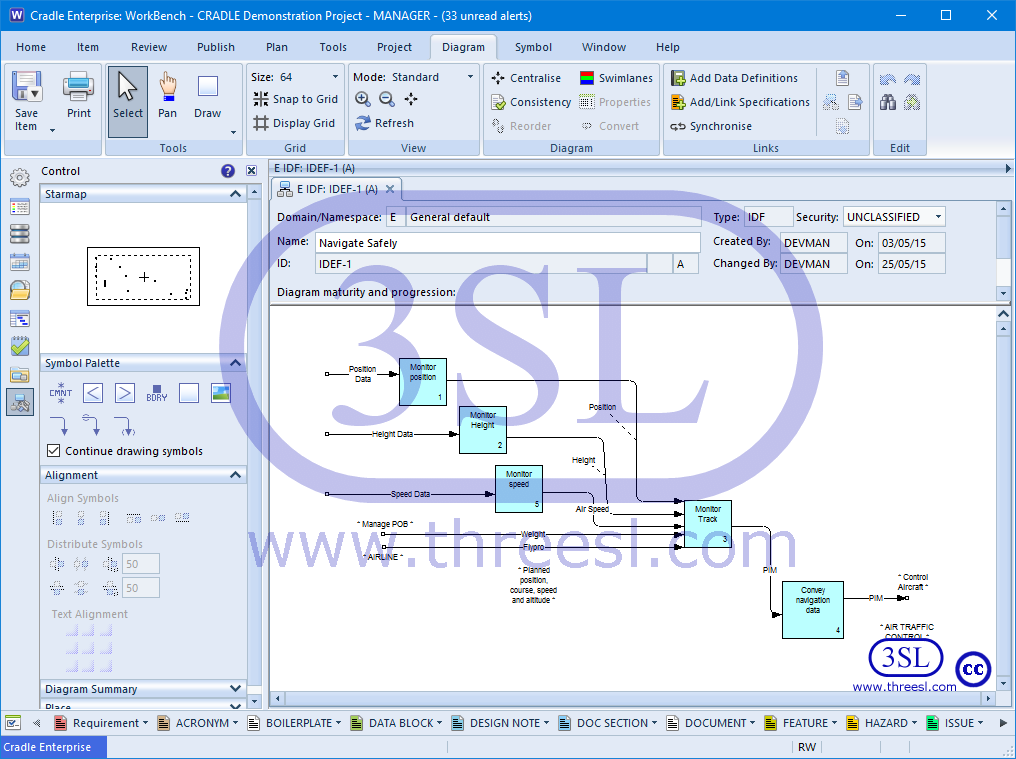IDEF0

Architectural Modelling: Ada Structure Graph (ASG)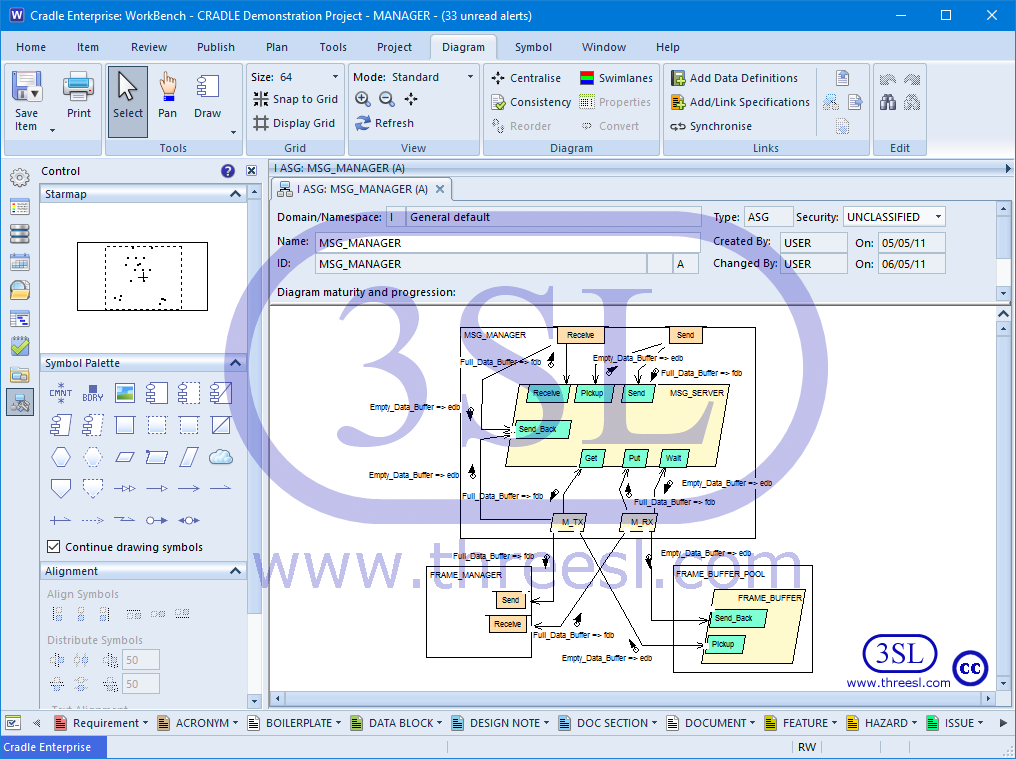Ada Structure Graph (ASG)

Architectural Modelling: Architecture Interconnect Diagram (AID)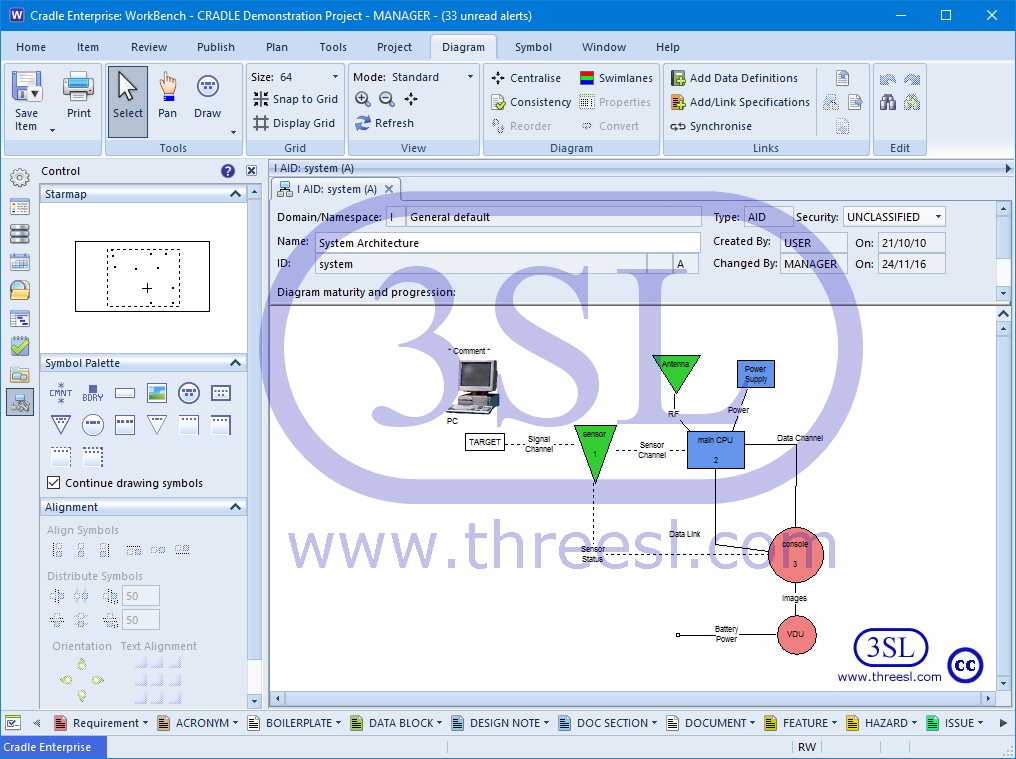Architecture Interconnect Diagram (AID)

Architectural Modelling: Physical Architecture Diagram (PAD)Physical Architecture Diagram (PAD)

Architectural Modelling: Software Architecture Diagram (SAD)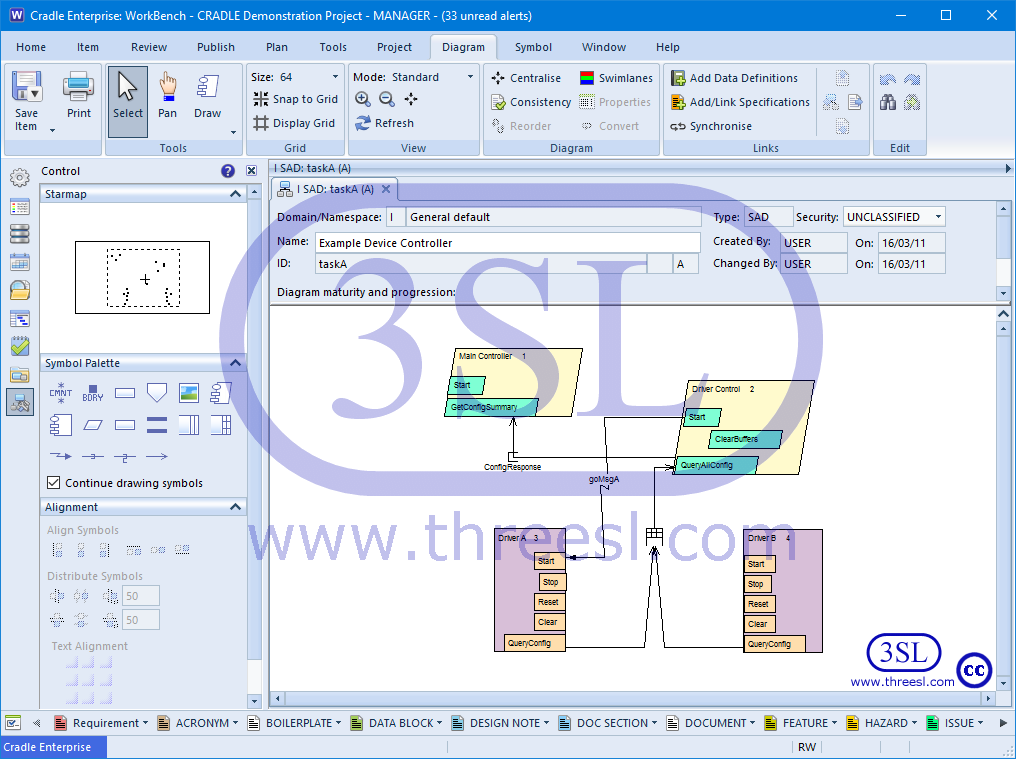Software Architecture Diagram (SAD)

Data Modelling: Entity Relationship Diagram (ERD)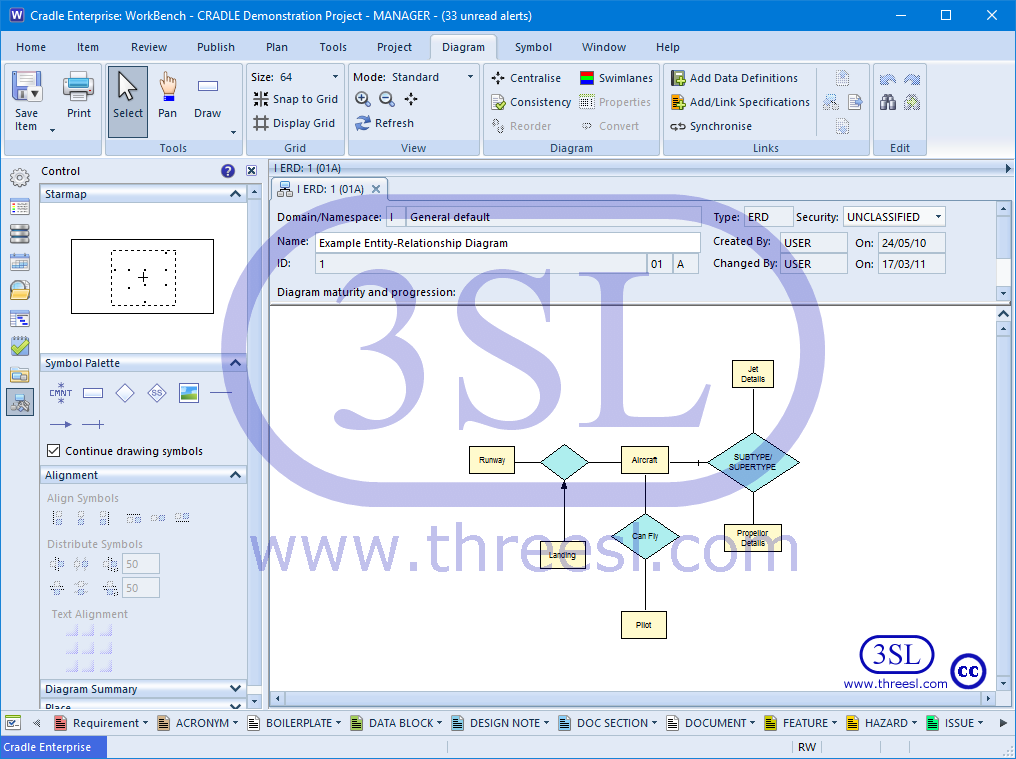Entity Relationship Diagram (ERD)

Data Modelling: Data Structure Diagram (DSD)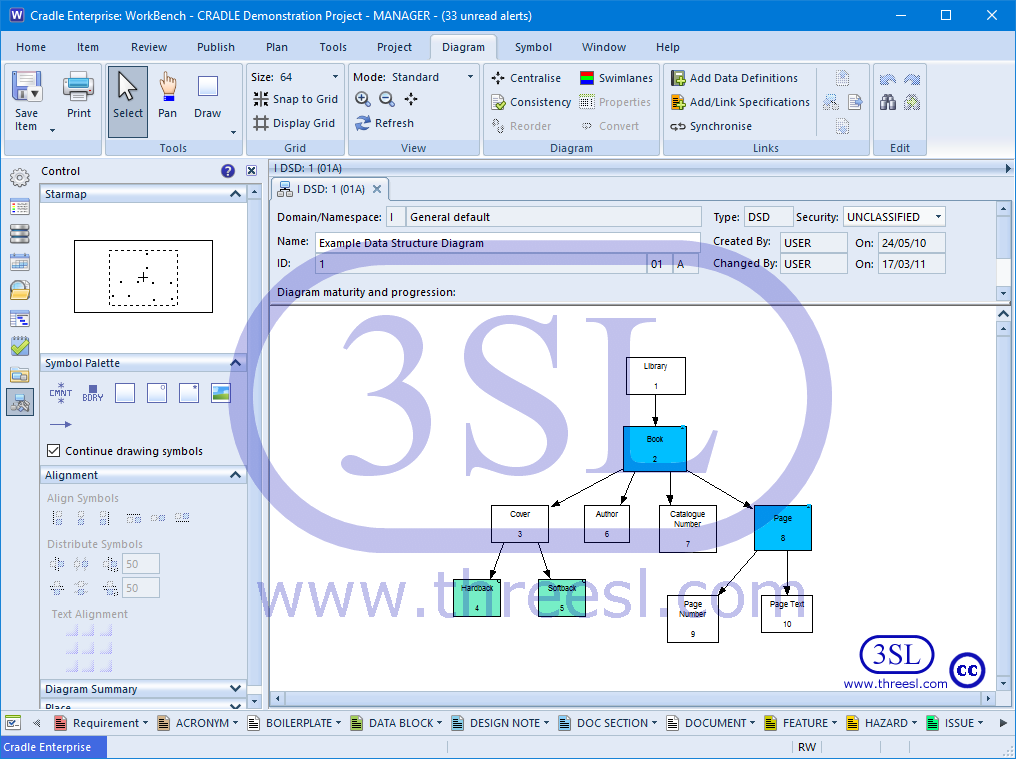Data Structure Diagram (DSD)

Behaviour Modelling: Extended Functional Flow Block Diagram (eFFBD)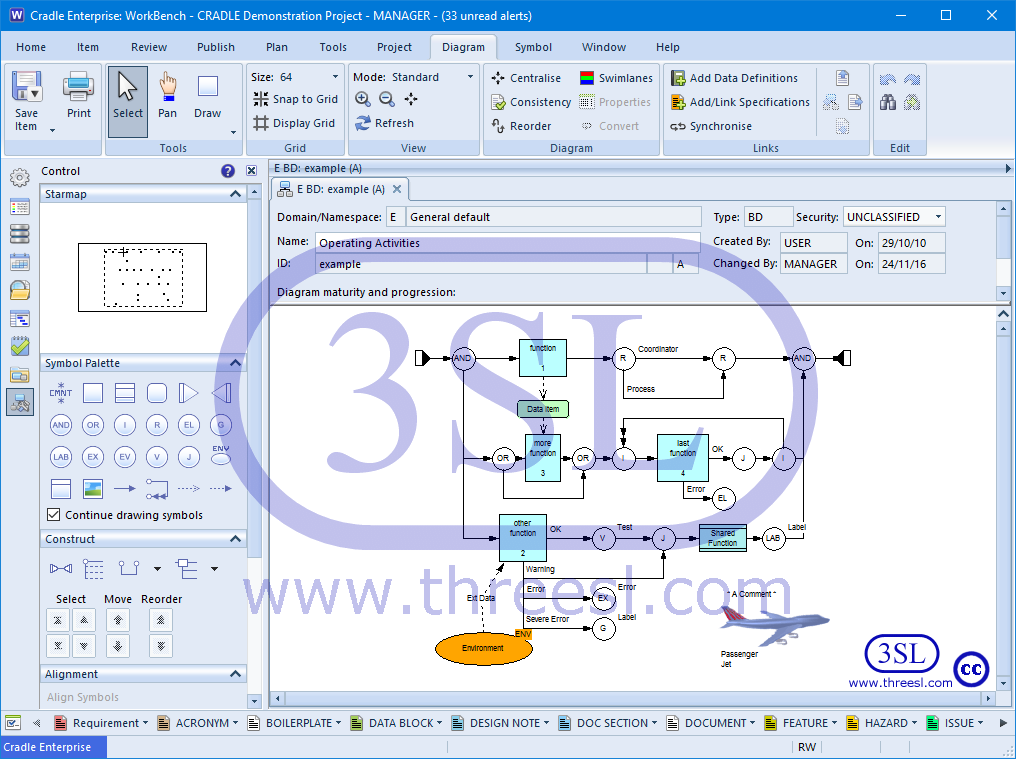Extended Functional Flow Block Diagram (eFFBD)

Process Modelling: Process Flow Diagram (PFD)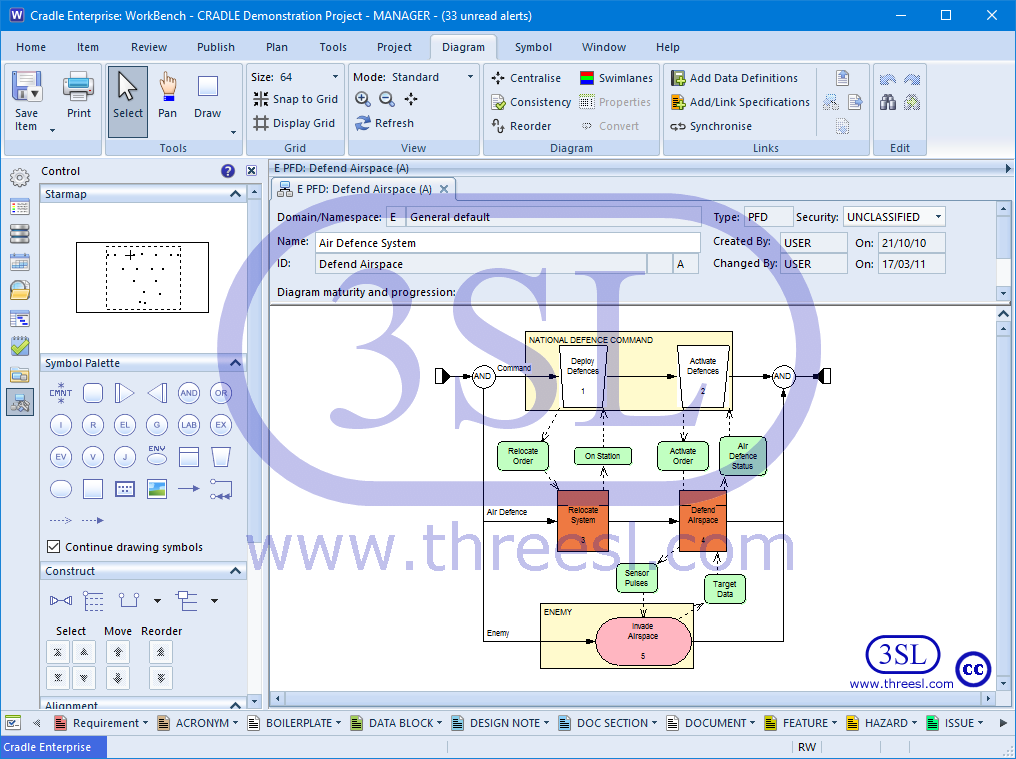Process Flow Diagram (PFD)

OO Modelling: Use Case Diagram (UCD)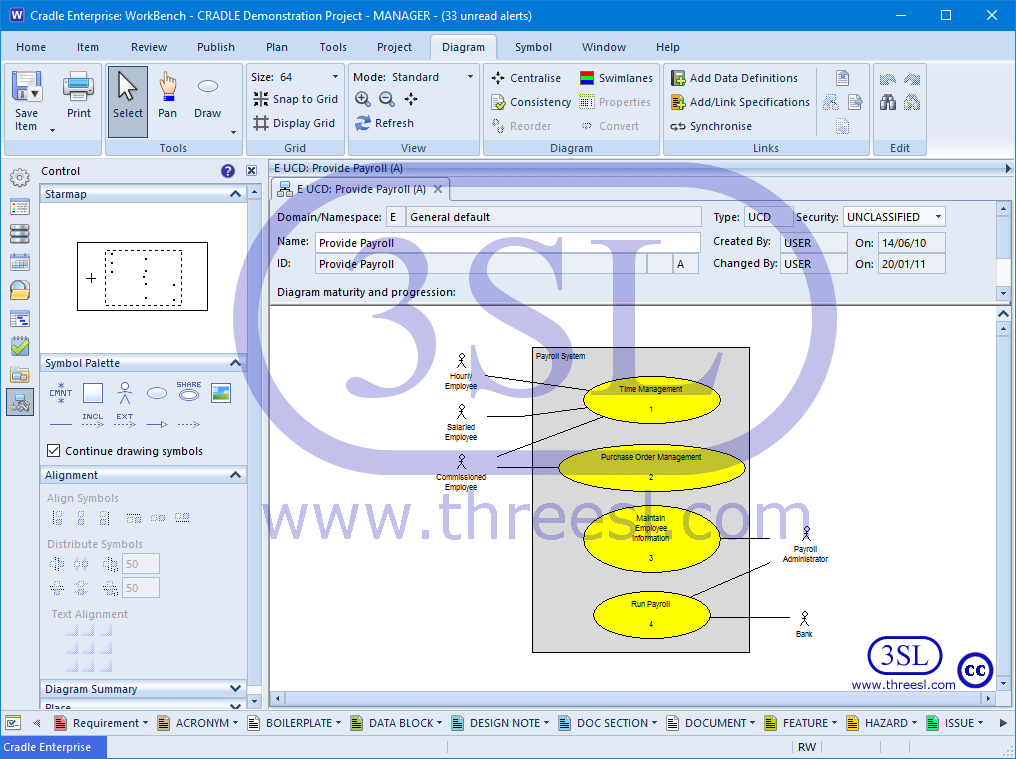Use Case Diagram (UCD)

OO Modelling: Package Diagram (PD)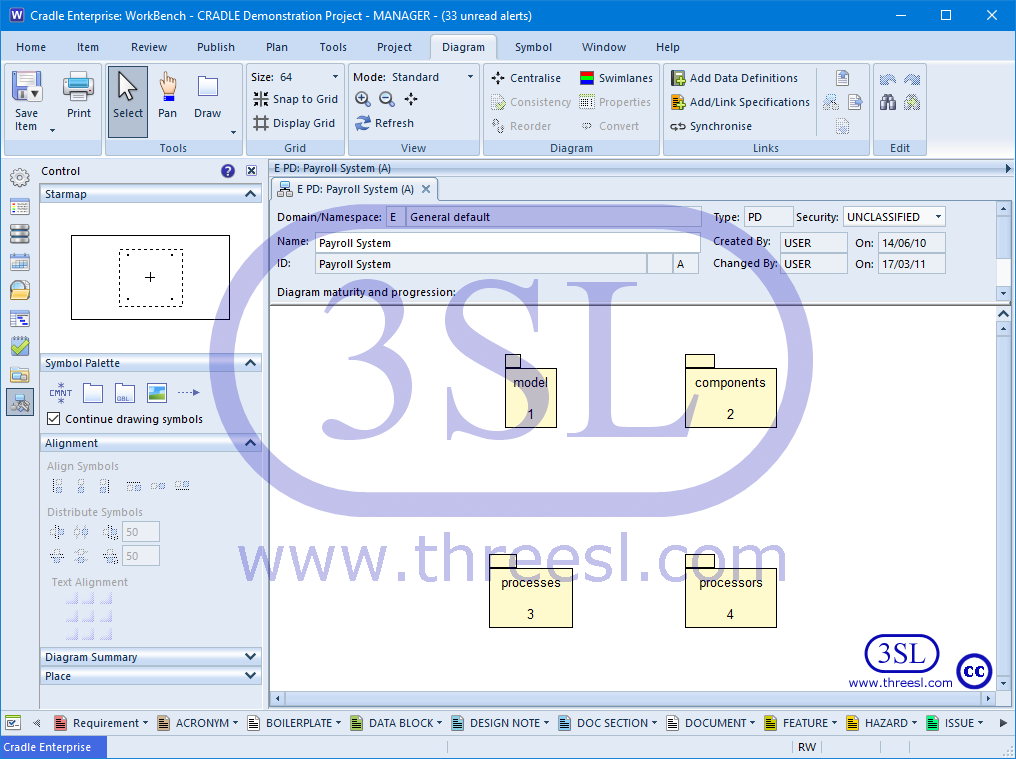Package Diagram (PD)

OO Modelling: Sequence Diagram (SQD)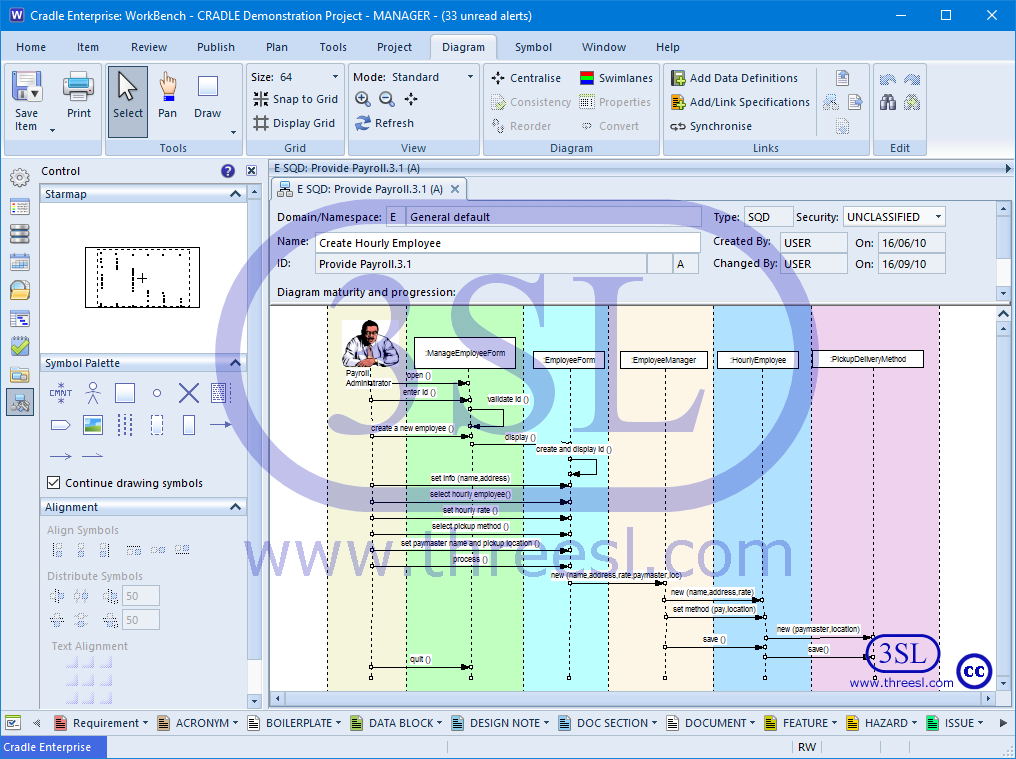Sequence Diagram (SQD)

OO Modelling: Collaboration Diagram (COD)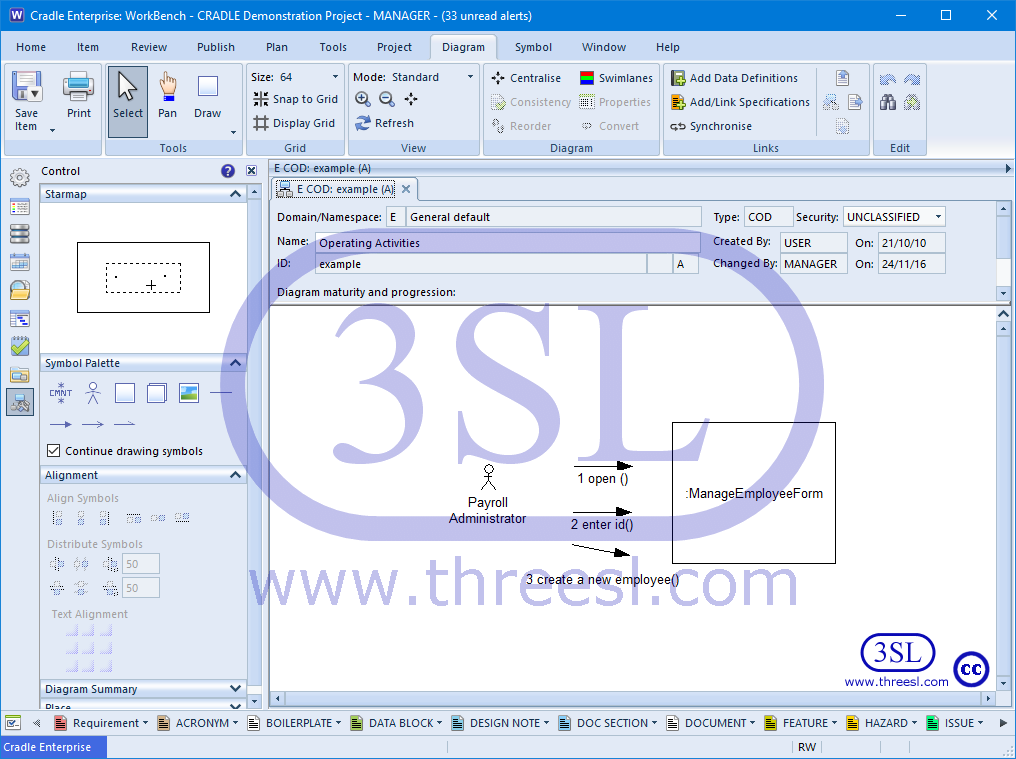Collaboration Diagram (COD)

OO Modelling: Class Diagram (CD)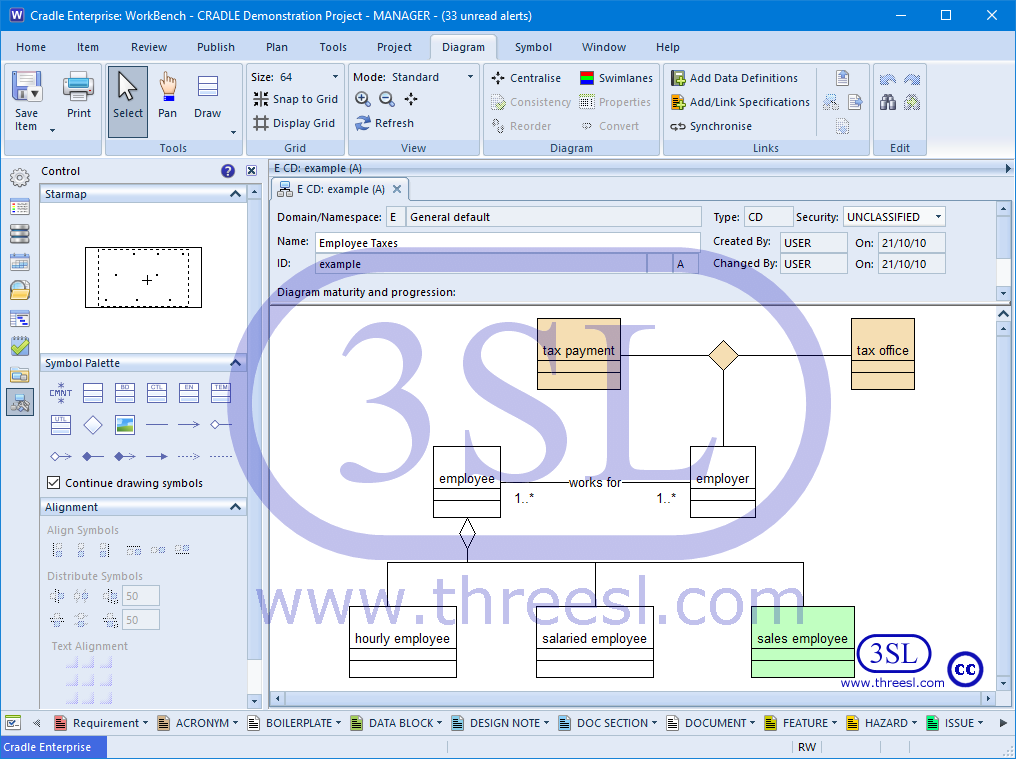Class Diagram (CD)

OO Modelling: Statechart Diagram (SCD)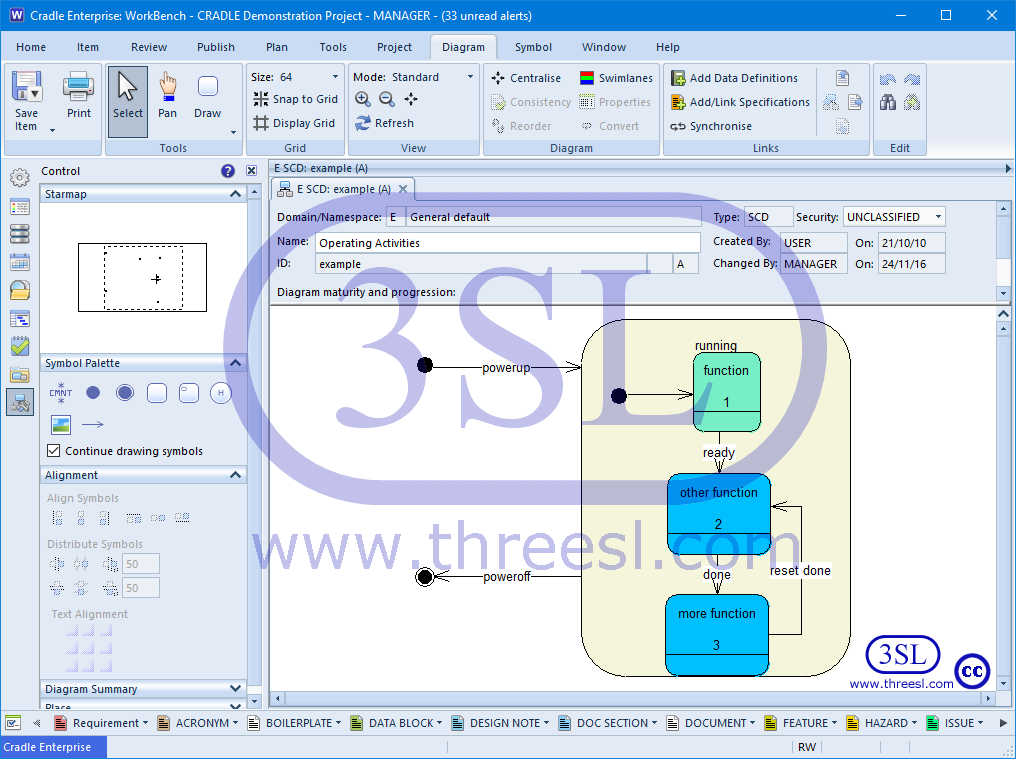Statechart Diagram (SCD)

OO Modelling: Activity Diagram (ACD)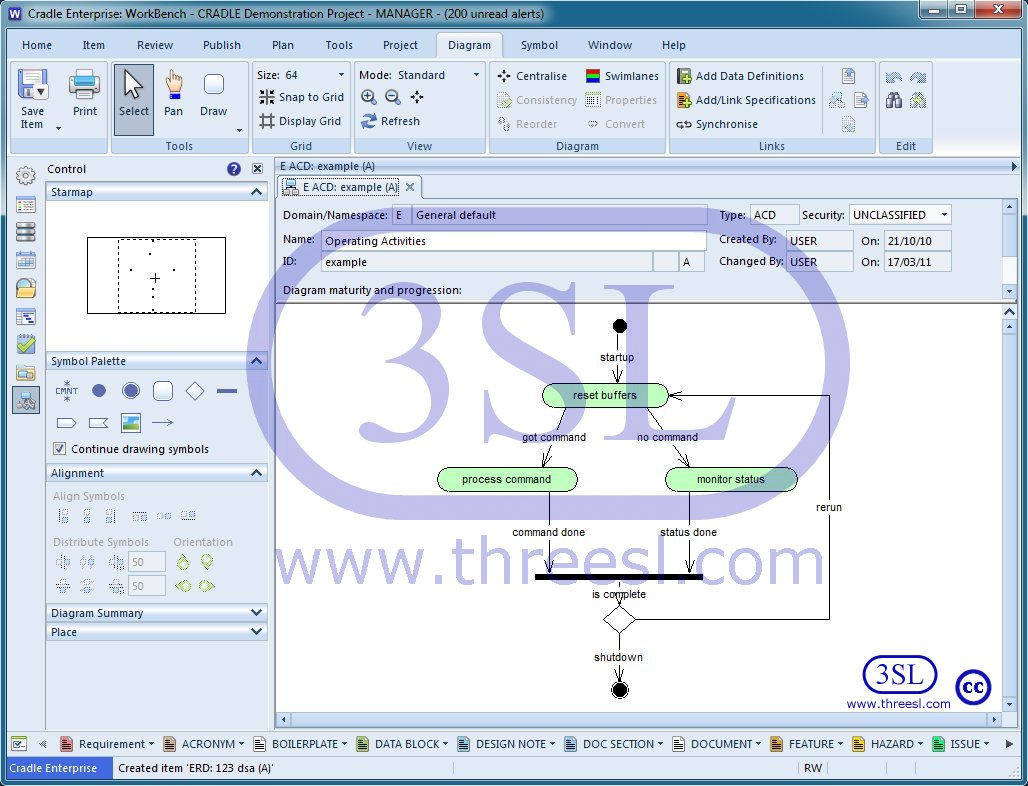Activity Diagram (ACD)

OO Modelling: Component Diagram (CPD)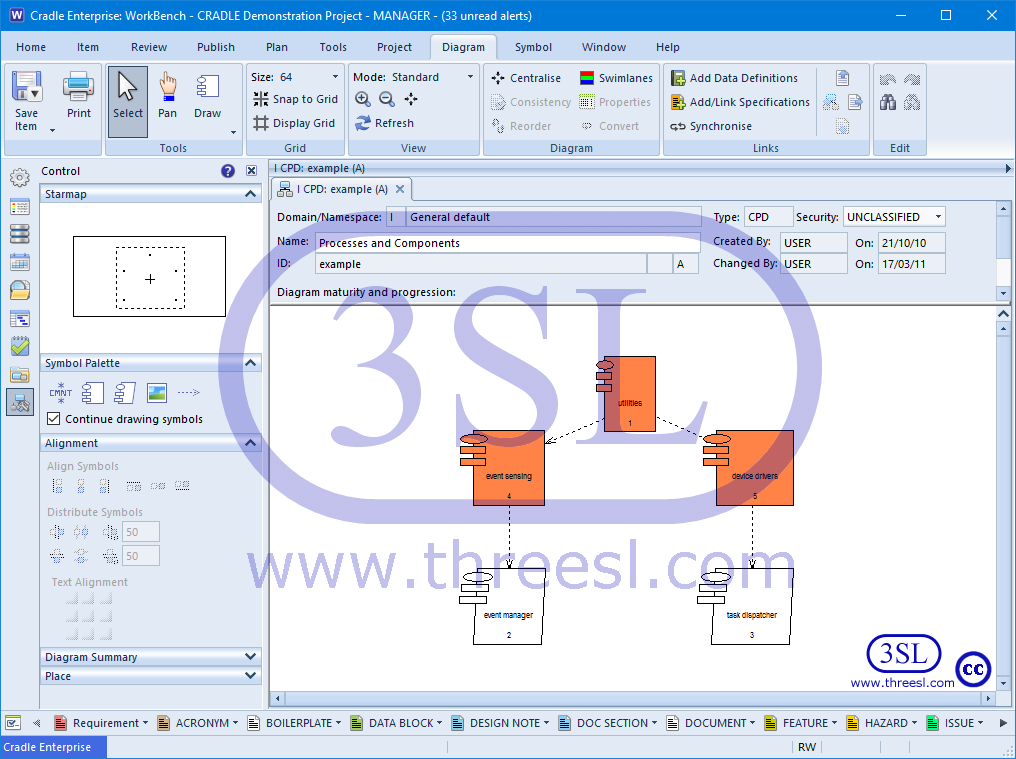Component Diagram (CPD)

OO Modelling: Deployment Diagram (DPD)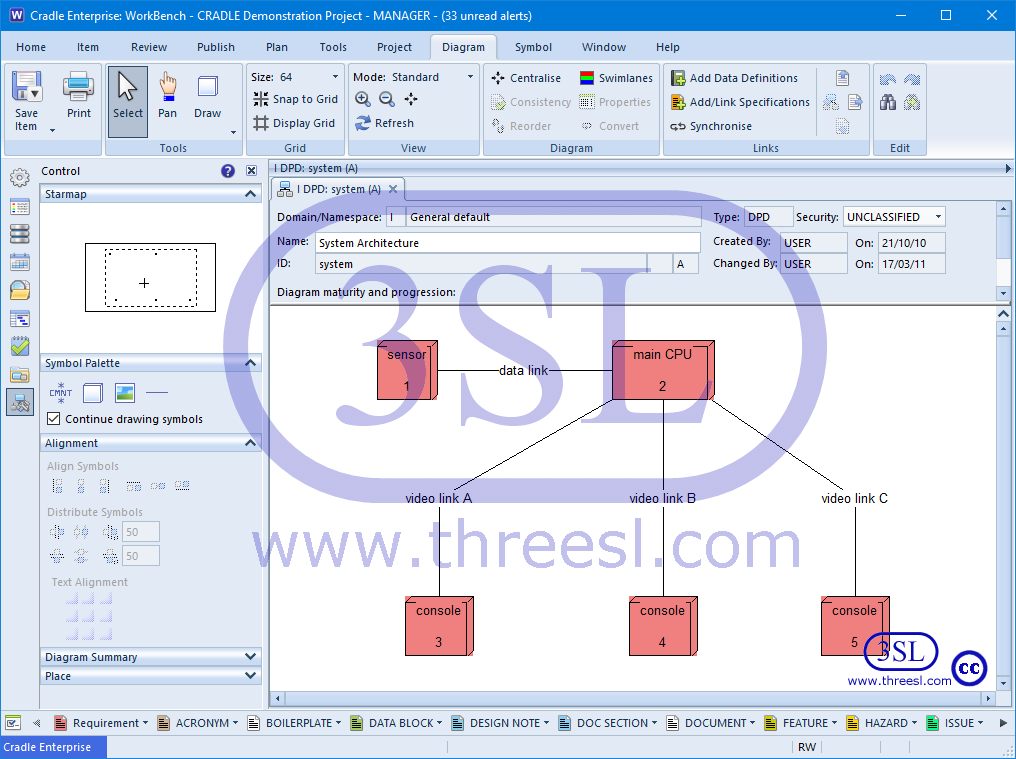Deployment Diagram (DPD)